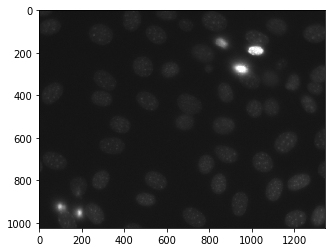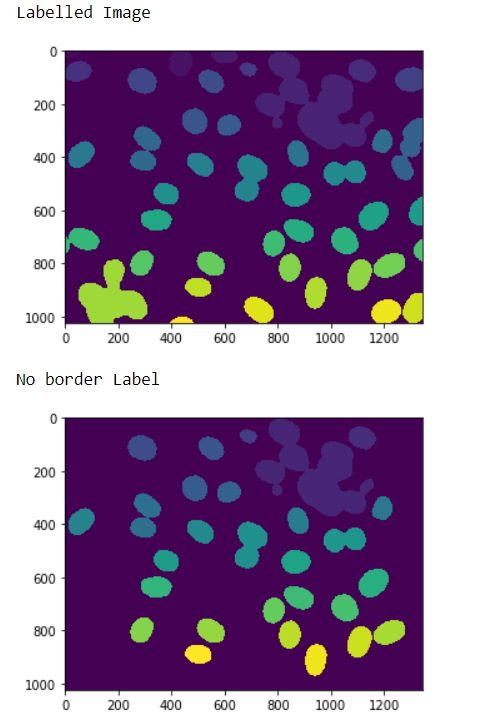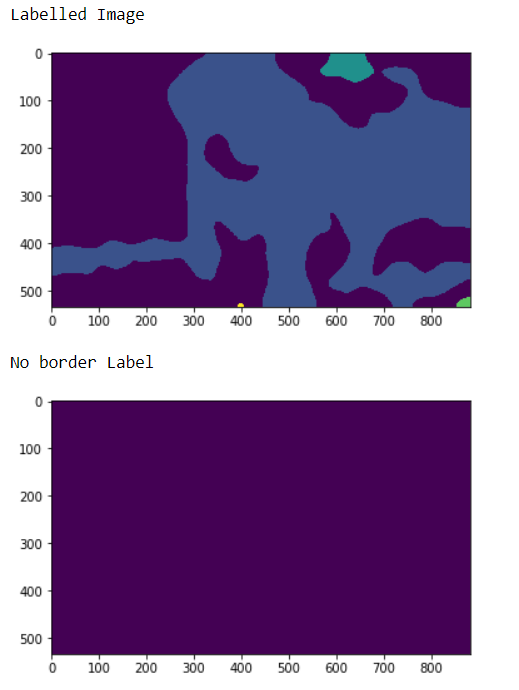# Mahotas – Removing Bordered Labelled

• Last Updated : 30 Jun, 2021

In this article we will see how we can remove the bordered label from the labelled image in mahotas. Border labels are those labels which are touching the border, we can create labelled image from normal image with the help of mahotas.label method.
For this we are going to use the fluorescent microscopy image from a nuclear segmentation benchmark. We can get the image with the help of command given below

`mhotas.demos.nuclear_image()`

Below is the nuclear_imageLabelled images are integer images where the values correspond to different regions. I.e., region 1 is all of the pixels which have value 1, region two is the pixels with value 2, and so on
In order to do this we will use mahotas.remove_bordering method

Syntax : mahotas.remove_bordering(labelled)
Argument : It takes labelled image object as argument
Return : It returns the labelled image without label at the borders

Example 1:

## Python3

 `# importing required libraries``import` `mahotas``import` `numpy as np``from` `pylab ``import` `imshow, show``import` `os` `# loading nuclear image``f ``=` `mahotas.demos.load(``'nuclear'``)` `# setting filter to the image``f ``=` `f[:, :, ``0``]` `# setting gaussian filter``f ``=` `mahotas.gaussian_filter(f, ``4``)` `# setting threshold value``f ``=` `(f> f.mean())` `# creating a labelled image``labelled, n_nucleus ``=` `mahotas.label(f)` `# showing the labelled image``print``(``"Labelled Image"``)``imshow(labelled)``show()` `# removing border labels``labelled ``=` `mahotas.labelled.remove_bordering(labelled)` `# showing the image``print``(``"No border Label"``)``imshow(labelled)``show()`

Output :Example 2:

## Python3

 `# importing required libraries``import` `numpy as np``import` `mahotas``from` `pylab ``import` `imshow, show` `# loading image``img ``=` `mahotas.imread(``'dog_image.png'``)``  ` `# filtering the image``img ``=` `img[:, :, ``0``]``   ` `# setting gaussian filter``gaussian ``=` `mahotas.gaussian_filter(img, ``15``)` `# setting threshold value``gaussian ``=` `(gaussian > gaussian.mean())` `# creating a labelled image``labelled, n_nucleus ``=` `mahotas.label(gaussian)`` ` `print``(``"Labelled Image"``)``# showing the gaussian filter``imshow(labelled)``show()` `# removing border labels``labelled ``=` `mahotas.labelled.remove_bordering(labelled)` `# showing the image``print``(``"No border Label"``)``imshow(labelled)``show()`

Output :My Personal Notes arrow_drop_up